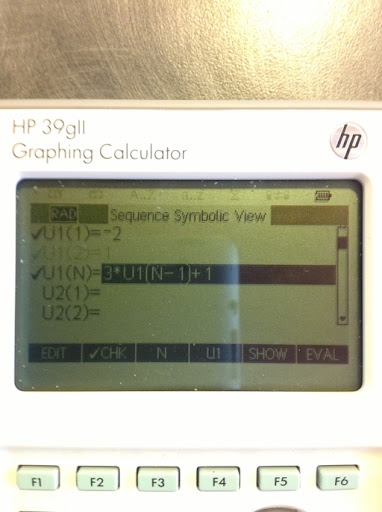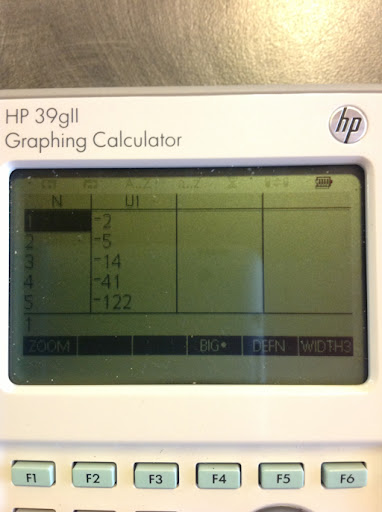## Saturday, February 9, 2013

### a_(n+1) = S * a_n + T

Hi everyone!

First a correction. In my Numerical CAS section, posted December 2012, I typed an extra quotation mark in the POLYBINE program for the Casio Prizm - that has been corrected. Much thanks to Ryan Maziarz who pointed that out to me.

Here is a link to the corrected post:
http://edspi31415.blogspot.com/2012/12/numeric-cas-part-2-binomial-expansion.html?m=1

Today we are finding the general formula for this recursion formula:

a_(n+1) = S * a_n + T

with the initial condition a_0 = A.

I could try using a characteristic polynomial, but with some observation we may be able to detected a pattern which leads us to a general formula.

Observe that:

a_0 = A

a_1 = S * a_0 + T
a_1 = S * A + T

a_2 = S * a_1 + T
a_2 = S * (S * A + T) + T
a_2 = S^2 * A + S * T + T
a_2 = S^2 * A + T * (S + 1)

a_3 = S * a_2 + T
a_3 = S * (S^2 * A + T * (S + 1)) + T
a_3 = S * A^3 + T * (S^2 + S + 1)

a_4 = S * a_3 + T
a_4 = S^4 * A + T * (S^3 + S^2 + S + 1)

From the pattern, observe that:

a_n = S^n * A + T * Σ(S^k, k=0, n-1)

With Σ(t^k, k=0, n-1) = (t^n - 1)/(t - 1) :

a_n = S^n * A + T * (S^n - S)/(S - 1)

An Example:

S = 3, T = 1, A = -2

Using the HP 39gii to generate the sequence (the 39gii uses a_1 as its initial term):The general formula is:

a_n = (3^n) * (-2) + 1 * (3^n - 1)/(3 - 1)
a_n = (-2) * 3^n + (3^n - 1)/2
with a_0 = -2. (The 39gii has u_1 = a_0)

Example:

a_2 = u_3 = (3^2) * (-2) + (9 - 1)/2 = -14

That is all for now, see you next time!

Eddie

This blog is property of Edward Shore. 2013

5 blog entries away from the 200th blog entry.

#### 1 comment:

1.Ensure Yourself Success By Using Printable GED Practice Tests
printable ged practice test free

### Retro Review: TI-95 Procalc

Retro Review:  TI-95 Procalc  This review covers the calculator itself, not any of the optional accessories which includes a ...